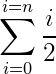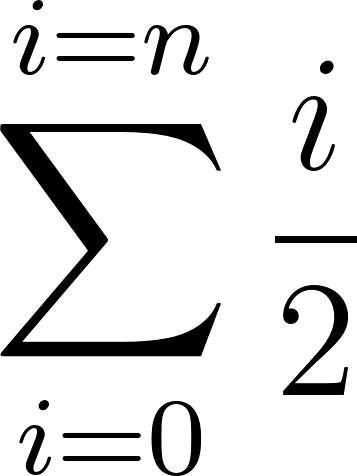gregwar /tex2png

LaTeX formula to PNG

17 388

0

60

7

17

v1.0.0 2015-09-20 12:56 UTC

This package is auto-updated.

Last update: 2022-01-23 22:11:58 UTC

This class provides a way to create easily LaTeX formulas.

With it, you can convert raw formulas like:

\sum_{i = 0}^{i = n} \frac{i}{2}

To nice images like:Requirement

To use this library you'll need :

• latex : to compile formulas (with math support)
• dvipng : to convert dvis to png
• shell_exec : you need to be able to call the php shell_exec() function

You'll also need a temporary folder and, of courses, enough permissions to write to the target directory

Usage

Just include the Tex2png.php file or register this repository directory as the Gregwar\Tex2png\ namespace and do the following :

<?php

// This will create a formula and save it to sum.png
Tex2png::create('\sum_{i = 0}^{i = n} \frac{i}{2}')
->saveTo('sum.png')
->generate();

You can have a look at the example in example/ directory.

Using the cache

Tex2png library includes a caching system allowing you to generate images only one time. To do so, just don't tell Tex2png what is the target file :

<?php

Tex2png::create('\sum_{i = 0}^{i = n} \frac{i}{2}')
->generate();

// The filename will be choosen using an hash of the formula and
// the image density. (file name will look like cache/tex/3/0/2/e/6/8febefe7aaed9eeb8abf09070d10e02e93e.png)

If the file already exists, it will not generate anything, else, it will generate it. This results in a tiny but powerful caching system avoiding to regenerate a formula PNG twice.

Note that you can change the cache directory calling the setCacheDirectory() function

This is based on the Gregwar/Cache library.

Changing the density

The second constructor/create() parameter is the image density :

<?php

Tex2png::create('\sum_{i = 0}^{i = n} \frac{i}{2}', 300)
->generate();

Default density is 155, you can choose to generate really big images, this is an example of the formula with a density of 1000 :